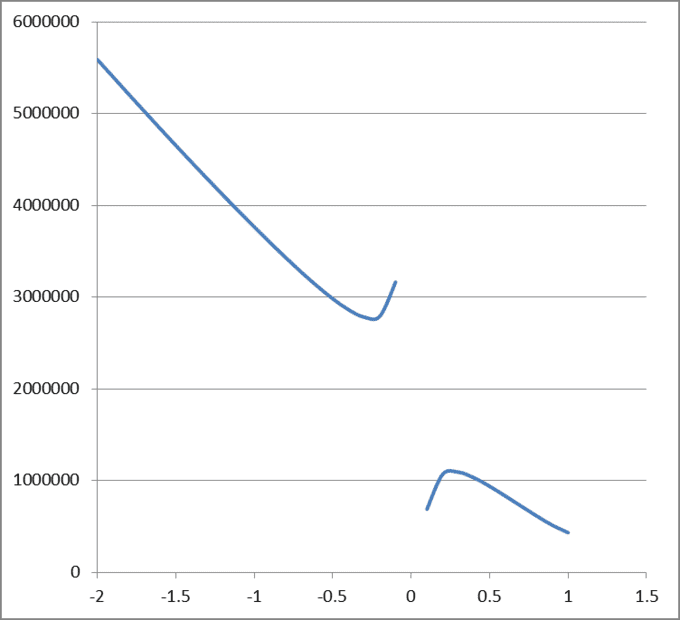# How to find the positive maximum value of a function

• Keysa

#### Keysa

TL;DR Summary
I want to find the positive maximum value of a function, but so far I always ended up with either a negative value or the value doesn't even show up.
This is the code that i wrote

Clear["Global*"]
Z = 500;
W = 100000;
G = 250;
H = 100;
K = 0.5;
T = 30;
L = 4000;
P = 5;
S = 2.5;
Y = 1;
A = 0.1;
V = 2.5;
J = 8000;
f[x_] := 1/
x {(J*Z*x*(2*Y - x))/(
2*Y) - ((W + T*G) + ((L + T*P)*2*Z*Y*(1 - ((Y - x)/Y)^1.5))/
3 + (H + T*S + A*L)*((
2*Z*Y*2*Y - 2*Z*Y*2*Y*((Y - x)/Y)^2.5 -
2*Z*Y*5*x*((Y - x)/Y)^1.5)/15))};
Plot[f[x], {x, -2, 2}]
FindMaximum[f[x], x]

Hay Keysa, what function and what domain? Can you write it in LaTeX? You use Python?

Hello Keysa,##\qquad##!​

It would help if you mentioned the language in which you try to do this.
If I use Excel () to plot your function, I getand an error if ##x\ge 1##.

You sure you have no typos in your very long expression ?

##\ ##

Some formatting and added parenthesis would help us to see what your equation is. I can't see how much is in the denominator. If @BvU's graph is correct, then the maximum depends on the range of x.

What I plotted is
Code:
f(x) = 1/x *((J*Z*x*(2*Y - x))/(2*Y) - ((W + T*G) + ((L + T*P)*2*Z*Y*(1 - ((Y - x)/Y)^1.5))/3 + (H + T*S + A*L)*((2*Z*Y*2*Y - 2*Z*Y*2*Y*((Y - x)/Y)^2.5 -2*Z*Y*5*x*((Y - x)/Y)^1.5)/15)))`

but, since I am not a computer nor a robot, I have a hard time chopping it up into digestable pieces.
Do you have a source, an original, or anything that helps sorting out your function ?

##\ ##